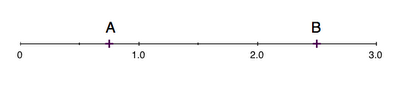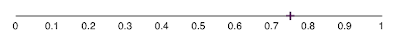## Wednesday, July 18, 2012

Inspired by the a segment on NPR about machinists. Here is a short lesson on adding and subtracting decimals. First, if you are not great at subtraction, here are a couple example problems to refresh.

First, what is a decimal? It is a way to locate a point between two whole numbers.

In the Figure 1 below A and B are decimals. First, you can see on the bottom that the numbers go from zero to three. We will start with B first. If you take a look closely you will see that right above 0, 1, 2, 3, and in between 0 and 1, 1 and 2, 2 and 3 there is a small mark, called a tick mark. B is right on the tick mark between 2 and 3. Since each number of the decimal is a way of counting from 0 to 9 with 5 being in the middle, B is at 2.5.Figure 1: A and B are Decimal Numbers
The thing about decimals is that little period matters. For example, 20.6 is much different than 2.06. That's 20.6 inches or 20.6 dollars instead of 2.06 inches or 2.06 dollars. Now let's take a look at A.Figure 2: Close Up of A
First you can see from the scale that the decimal gets one number higher each time you move from left to right. Instead of counting 1, 2, 3, 4, 5, 6, 7, 8, 9, 10 you could count 0.1, 0.2, 0.3, 0.4, 0.5, 0.6, 0.7, 0.8, 0.9, 1.0. Since A is half way between 0.7 and 0.8 we will call it 0.75.

Now the big question is: how far is it from A to B?

We know that A is at 0.75 and B is at 2.50.

Subtract A from B:

2.50 - 0.75 = ?

This can also be shown vertically so that the decimal goes strait down:

2.50         Start with the numbers on the right 0 - 5 = ?
- 0.75         Here we need to carry the one so make the 2.50 a 2.4 (10).
= ?.??

2.4(10)
- 0.75        10 - 5 = ?   Answer on the right side: 5
= ?.??        Next we do the middle 4 - 7 = ? We need to carry the one again.

1.(14)       Make the 2 a 1 and the 4 a 14 so instead of 2.4 you have 1.(14).
- 0.7           14 - 7 = ?   Answer in the middle just to the right of the decimal: 7
= ?.?5

1                Finally we do the left side 1 - 0 = ? Answer on the right: 1
- 0
= ?.75           In total the answer is: 1.75

What if we wanted to add A and B?

+ 0.75         Then in the middle 5 + 7 = ? Here we will need to carry the one.
= ?.??         The answer is 12 so leave the 2 down at the answer
Add one to the right side

2 (+1)       Here we have 2 + 1 = 3 and take that and add 0
+ 0              3 + 0 = ? Answer: 3
= ?.25         In total the answer is: 3.25

Now for the real life example!Figure 3: Decimals Used in a Manufacturing Drawing
Now we will look at Figure 3. This is the test question. This is from an actual manufacturing drawing. Machinists look at this kind of drawing all the time. The question is, how long is the slot on the left?

First we need to find the end points of the slot. On the left side we see that the left side is at 5.75 (inches from the right) and the right side is at 4.00 (inches from the right) by following the lines to their dimensions. We need to use subtraction here. Using the big number and subtracting the small number will give us the difference between the start and stop for that slot.

5.75 in.
- 4.00 in.
= 1.75 in.

And there you have it, how to add and subtract decimals. When there are units like inches (in.) or millimeters (mm) or some other length given it is good practice to always write down the units beside your calculations. NASA crashed into a planet once because parts of the spacecraft were designed by two different groups that used different units. The wrong units can be an expensive mistake.